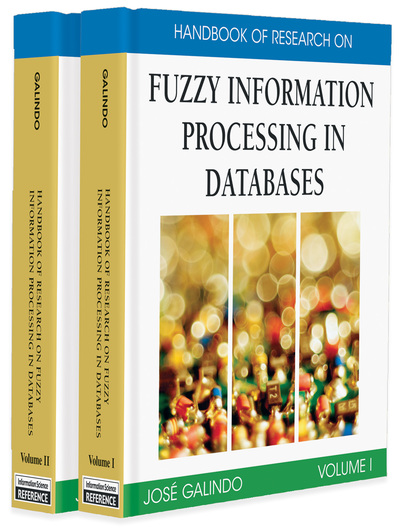# Data Model of FRDB with Different Data Types and PFSQL

Aleksandar Takaci, Srdan Škrbic
DOI: 10.4018/978-1-59904-853-6.ch016
OnDemand:
(Individual Chapters)
Available
\$29.50
No Current Special Offers

## Abstract

This chapter introduces a way to extend the relational model with mechanisms that can handle imprecise, uncertain, and inconsistent attribute values using fuzzy logic. It describes details on how the relational model is extended in order to include fuzzy capabilities. In addition, we describe a query language called PFSQL for this fuzzy database model. Besides basic fuzzy capabilities, this query language adds the possibility to specify priorities for fuzzy statements. This appears to be the first implementation that has such capabilities. Also we describe the relations on FRDB (fuzzy relational database) and PFCSP (priority fuzzy constraint satisfaction problems) and GPFCSP (generalized priority fuzzy constraint satisfaction problems), theoretical concepts vital for the implementation of PFSQL. The authors propose several points in which this research and implementation can be continued and extended, contributing to better understanding of fuzzy database concepts and techniques and giving numerous possibilities for further development in this area.

## Key Terms in this Chapter

PFCSP (Priority Fuzzy Constraint Satisfaction Problem): An expansion of FCSP by adding an importance degree to each of the constraints. In that manner, besides a satisfaction degree, each constraint has its importance value, its priority. They make decisions that depend not only on the satisfaction degree of each constraint (which is the case in FCSP), but also on the priority that each constraint has and are introduced by an axiomatic framework.

FRDB (Fuzzy Relational Database): A relational database with model extended by the mechanism that can handle imprecise, uncertain, and inconsistent attribute values using fuzzy logic.

GPFCSP (Generalized Priority Fuzzy Constraint Satisfaction Problem): An expansion of the PFCSP introduced in order to achieve the formal ground for PFSQL. PFCSP axiomatic framework is expanded by introducing negation and disjunction. For the conjunction, a t-norm and for disjunction the dual of the t-norm is chosen. Negation has to be a standard one.

Priority Fuzzy SQL (PFSQL): A variation of SQL language extended with fuzzy capabilities with an option to assign priorities to query conditions.

Fuzzy Quantity: Normalized fuzzy set with either increasing or decreasing membership function; that is, their kernel is bounded from either the left or the right, and unbounded from the other side. Linear fuzzy quantities are used to describe notions like “tall people,” “small salary,” and so forth.

Linguistic Label: Linguistic labels are named fuzzy values from the domain. They are used to represent most common and widely used expressions of a natural language (such as “tall people,” “small salary,” or “mediocre result”).

FCSP (Fuzzy Constraint Satisfaction Problem): An expansion of CSP (Constraint Satisfaction Problem) by allowing a constraint to have a satisfaction degree from the unit interval, that is, allowing many levels of constraint satisfaction. Constraints can be modeled as fuzzy sets over a particular domain and the degree of satisfaction of a constraint is the membership degree of its domain value on the fuzzy set that represents it.

## Complete Chapter List

Search this Book:
Reset